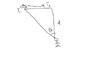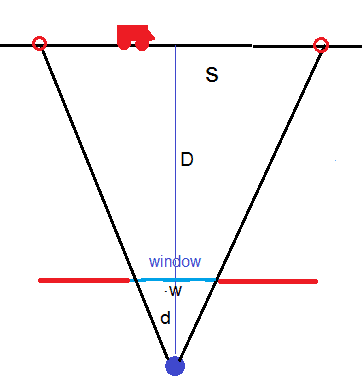# Calculus derivatives word problem

• robin123

## Homework Statement

Is it possible to accurately approximate the speed of a passing car while standing in the
protected front hall of the school?
Task: Determine how fast cars are passing the front of the school. You may only go
outside to measure the distance from where you are standing to the road. All
other measurements must be taken from inside the building (I want you to stay
warm and dry!). Make sure to clearly explain your process to gauge the speed of
passing cars and submit all of your work!

## Homework Equations

speed= distance/time

## The Attempt at a Solution

time can be calculated for distance we have to suppose an angle and working with triangles but i am not able to figure out how

<Moderator's note: Approved as OP doesn't ask for a solution but just a guidance.>

Last edited by a moderator:

## Homework Statement

Is it possible to accurately approximate the speed of a passing car while standing in the
protected front hall of the school?
Task: Determine how fast cars are passing the front of the school. You may only go
outside to measure the distance from where you are standing to the road. All
other measurements must be taken from inside the building (I want you to stay
warm and dry!). Make sure to clearly explain your process to gauge the speed of
passing cars and submit all of your work!

## Homework Equations

speed= distance/time

## The Attempt at a Solution

time can be calculated for distance we have to suppose an angle and working with triangles but i am not able to figure out how

<Moderator's note: Approved as OP doesn't ask for a solution but just a guidance.>

Draw a section of the road and the perpendicular line from where you are standing to the road. That's the distance you know. With a surveyor's transit measure the angles to the starting and ending point where you can see the cars, and draw those two lines. Now use trigonometry and a timer.

Last edited:
•berkeman
With a surveyor's sextant measure the angles to the starting and ending point where you can see the cars,
Or even use just your protractor from your Trigonometry class, but it won't be as accurate. For extra credit, estimate your error when using the protractor versus using a super-accurate instrument to measure the angles to the first and last positions of the cars...@robin123 a similar problem, but with one less step (no speed involved) is how would you figure out the height of a flagpole with a protractor, a long tape measure, and no ladder.

•berkeman
Draw a section of the road and the perpendicular line from where you are standing to the road. That's the distance you know. With a surveyor's sextant measure the angles to the starting and ending point where you can see the cars, and draw those two lines. Now use trigonometry and a timer.
will there be 2 lines with different angles? does that mean i will have two diagrams?

will there be 2 lines with different angles? does that mean i will have two diagrams?
Just show us a sketch of your building, the road, the distance to the road, and the angles to the initial and final car positions as it passes by. Use the Upload button at the lower right of the Reply window to Upload a PDF or JPEG of your sketch.

Just show us a sketch of your building, the road, the distance to the road, and the angles to the initial and final car positions as it passes by. Use the Upload button at the lower right of the Reply window to Upload a PDF or JPEG of your sketch.
will it look like this?

#### Attachments

•screenshot_20180517_223236.jpg
7.7 KB · Views: 331
Yes. You could measure on the other side of the perpendicular too until the car gets out of sight. Do you know how to use trigonometry to figure out how far the car goes while it is in sight and you are timing it?

•berkeman
Yes. You could measure on the other side of the perpendicular too until the car gets out of sight. Do you know how to use trigonometry to figure out how far the car goes while it is in sight and you are timing it?
yeah, I can use the trigonometry but for that other side, I need an angle. I am confused about that angle. part. For the time I will start with 0s and till the time I can see it.

#### Attachments

I would do it with two angles. The angle ##\theta## you have drawn for the car approaching the perpendicular and another angle ##\alpha## on the other side of the perpendicular as the car leaves the perpendicular. Those angles aren't zero. They are what you are going to measure so they are known.

I would do it with two angles. The angle ##\theta## you have drawn for the car approaching the perpendicular and another angle ##\alpha## on the other side of the perpendicular as the car leaves the perpendicular. Those angles aren't zero. They are what you are going to measure so they are known.
You do not need to measure angles . Measure the distance between the road and the wall, D. Stand at a distance d from the window of your room. Measure the width of the window: w. Measure the time you see the car passing in front of the window. The triangles shown are similar, so you can calculate the distance s the car travels from the measured data: D, d, and w.#### Attachments

@ehild: Aren't you assuming the building and window are parallel to the road?

@ehild: Aren't you assuming the building and window are parallel to the road?
Yes, I assume. It is quite common, that the buildings are parallel to the road isn't it?
Otherwise the problem would be tricky to measure angles. And the distance of the road from the building would not be constant along the route of the car.

You do not need to measure angles . Measure the distance between the road and the wall, D. Stand at a distance d from the window of your room. Measure the width of the window: w. Measure the time you see the car passing in front of the window. The triangles shown are similar, so you can calculate the distance s the car travels from the measured data: D, d, and w.
View attachment 225873
i am confused that how D,d,w can help us calculate s

View attachment 225877
The AOB triangle is similar to POQ triangle. What is the ratio s/w in terms of d and D?
if the above two triangles are similar, that's mean s should be equal to w?

if the above two triangles are similar, that's mean s should be equal to w?
NO. They are not congruent. What does similar mean in geometry?

Sides are proportional
Which sides are proportional?

S is the length taken by the car. It is the base of the big triangle.
W is the width of the window, it is the base of the small triangle.
D is the distance of the road from the wall. (Measured)
d is your distance from the wall inside the building (also measured) .
The height of the small triangle is d.
What is the height of the big triangle?
The ratio of heights of the triangles is the same as the ratio of the bases. So what is s/w in terms of d and D?

S is the length taken by the car. It is the base of the big triangle.
W is the width of the window, it is the base of the small triangle.
D is the distance of the road from the wall. (Measured)
d is your distance from the wall inside the building (also measured) .
The height of the small triangle is d.
What is the height of the big triangle?
The ratio of heights of the triangles is the same as the ratio of the bases. So what is s/w in terms of d and D?
in that case, we can find S by s/w=D/d we have three things given and we have to find S

Last edited:
So, what formula do you get for the speed of the car using the window method?
Also, since you said you can do the trig, what formula do you get using the one or two angles method mentioned earlier?

So, what formula do you get for the speed of the car using the window method?
Also, since you said you can do the trig, what formula do you get using the one or two angles method mentioned earlier?
isn't the formula still the same that speed=distance traveled by car / the time it took to travel that distance
if I have the angle I can just use the height and tan(theta) to figure out the distance traveled by car.
s/d=tan(θ)

Last edited: